# A Hybrid Privacy Preserving Scheme Using Finger Print Detection in Cloud Environment

A Hybrid Privacy Preserving Scheme Using Finger Print Detection in Cloud Environment

Bennett University, Greater Noida, India

Corresponding Author Email:
garimaverma.research@gmail.com
Page:
343-351
|
DOI:
https://doi.org/10.18280/isi.240315
6 April 2019
|
Accepted:
10 June 2019
|
Published:
31 August 2019
| Citation

OPEN ACCESS

Abstract:

Cloud computing provides a new paradigm of computing. It offers a scalable, manageable and huge pool of resources that can be accessed by users from anywhere anytime. It also ensures the integrity of data stored on the cloud. But ensuring the confidentiality and integrity of sensitive information is still a big challenge. To overcome this challenge, a hybrid two-phase security system for preserving the privacy of data on the cloud has been proposed. The hybrid approach combines feature extraction and encryption techniques to enhance the security of accessing data from the cloud. At first, the minutiae point has been extracted from the biometric fingerprint, locally collected from the state university in Northern India. The private key has been finalized by generating an elliptic curve using the minutiae point for achieving better encryption of fingerprint. The effectiveness of the approach has been tested in terms of similarity score, False Matching Ratio (FMR), False Non Matching Ratio (FNMR) and recognition accuracy, when applied on the local fingerprint database. The evidence of the outcomes suggests that the proposed technique ensures relatively improved security and privacy of data in the cloud system as compared to some recent state-of-art methods.

Keywords:

cloud computing, security, biometric, fingerprint detection, minutiae points, elliptic curve

1. Introduction

Biometrics identification is one of the most popular methods used nowadays for identifying the authenticity of an individual. All methods of biometric identification such as face recognition, iris, etc. have their own uniqueness , , such as two persons cannot have same fingerprint, and persistence. As biometric features usually do not change over time and age, so biometric-based recognition systems are being focused day by day for identification and security of data on the cloud. These biometric systems perform user authentication by verifying an individual's characteristics. For this, it is required to maintain the database of biometric features of all individuals. Whenever any user wants to access data or resources, first, the process of verification starts. In the verification process, a user’s biometric features are matched with the stored template in the database using any matching technique .

All biometric methods are generally categorized into two categories 1) Physical, 2) Behavioral . Physical biometric methods are a fingerprint, palm print, iris identification, retinal scanning, face recognition, etc. while the behavioral biometric methods are DNA matching, voice recognition, signature, handwriting, etc. . All type of biometric characteristics is unique and measurable for identification and verification of an individual .  There are various advantages of using biometric authentication as compared to conventional techniques of verification like cryptography. Some of the advantages of using biometric authentication are as listed below:

(1) Biometric methods give the category of authentication called as something you have. A person need not remember or carry identification separately like smart card, password, etc.

(2) Techniques are diminutive chances of stealing.

(3) Techniques are cost effective and accurate.

Techniques are easy, user-friendly, and secure , .

Nowadays, many of the smart devices like phones, laptops, doors, etc. are using an authentication mechanism based on biometric techniques instead of a simple password or swap cards or token system . There are very fewer chances to break the system unlike other traditional methods because every individual has unique biometric features and patterns  Due to all the above reasons, biometric authentication systems are reliable and suitable for cloud access also. The biometric data of all the users who access the cloud can be stored and verified at the time of cloud utilization. The safety of data, especially sensitive data like biometric data or other data and managing privacy preservation are the biggest challenge in the cloud computing systems . The popularity of cloud computing has forced researchers and developers to handle this issue very carefully. This can be achieved by the encryption of data available on the cloud, importantly biometric data, which will provide better security and privacy protection . In this paper, a novel biometric-based system using a fingerprint detection technique has been proposed for better privacy preservation and security in cloud systems. Biometric image templates are encrypted using the elliptic curve with the digital signature encryption algorithm. For providing better security and privacy on the cloud system the paillier algorithm has been used. The main advantage of using the paillier algorithm is its Homomorphic encryption properties . The road map of this paper is presented as follows. Section 2 discusses the related work done in the past by various researchers. Section 3 presents the proposed work. Section 4 describes the results generated from experiments and at last section 5 draws the conclusion of the work.

2. Literature Review

A lot of research has been done in the past to make secure cloud computing systems using various techniques. In most of the time, researchers have used the traditional cryptography techniques for providing security and privacy of data in the cloud. The main hassles with these techniques were in handling of security keys and data. For example, if the passwords are used for authentication of users then he may have a problem of remembrance. Especially if a user has several types of accounts then setting many passwords and remembering all these passwords is a hard task. Some other situations may arise like, if a user puts the same password for all his accounts, then it will provide a possibility of hacking all accounts. If the password is hacked or if the user saves the password in some file, then all accounts will be hacked if that file is hacked. To avoiding the situation of remembrance of password, smart cards can be used but, which have to be carried by the user all the time. If anytime it is Lost or stolen, then it may push users to some critical situations that can be considered as a major drawback of using smart cards. The above stated problems can be solved up to a great extent with biometric authentication due to its most important property i.e. “something that you have”.

Literature reveals that Bhattasali et al,  surveyed various biometric techniques in their work. Authors claimed that remote accessing of any type of data using biometric systems is more challenging in comparison to access from a local place. In these situations, it is unavoidable to prevent unauthorized access. Biometric authentication systems are more efficient in comparison to the traditional system of authentications. Naveed et al,  analyzed the various biometric authentication techniques in the cloud computing environment and explores how these techniques could help in reducing security threats. The privacy reserving cloud-based system with biometric identification has been proposed by Haghighat et al, .  Authors have used k-d tree approach to create encrypted queries for preserving data secure. In the year 2016, Hahn et al,  proposed an effective privacy preserving fingerprint identification scheme for cloud computing systems with a homomorphic encryption scheme. The authors tested the proposed scheme on the Amazon EC2 cloud. In the year 2018, Bala et al,  presented a biometric-based homomorphic encryption algorithm for data transmission in cloud systems. The proposed scheme was able to handle phishing and shoulder surfing attacks in the cloud environment. In a study done by Pan et al,  authors said that biometric identification provides lots of convenience to users of cloud computing systems but simultaneously increases privacy concerns also. In this study, researchers have studied various attacks and also validated them in a cloud environment. Kumar  et al. , proposed a security scheme using face recognition biometric identification approach in their proposed scheme on the cloud computing environment. As the main focus of the proposed work is on cloud security and privacy, so literature survey of security-oriented research papers has been continued. Lee et al. , analyzed the benefits of fingerprint identification in comparison to other biometric forms. The author has also discussed various case studies of companies in the UK, to justify his work and proved that the fingerprint identification system is comparatively better than other biometric systems. Zhang et al.  proposed a new privacy-preserving scheme based on biometric identification which ensures lightweight database computations. They have designed a biometric data encryption algorithm and introduces perturb terms in biometric data. The biggest challenge in cloud systems is to provide an efficient solution for security that gives access to resources and data which are outsourced to the cloud. To overcome this issue, Kumari et al. , devised a biometric authentication system for the multi-cloud server. They have used the bio-hashing technique for better accuracy of pattern matching. Al et al. , addressed security issues of mobile cloud computing by presenting an effective model to solve the identification problem in the mobile cloud using fingerprints. They have combined fingerprints with a password to make the system much strong. Shakil et al. , proposed the biometric authentication system for the health care database by introducing a signature-based system. with the help of a back-propagation network. Encouraged by the stated techniques, one hybrid approach in combination with the biometric and encryption technique has been proposed to preserve better security as well as privacy in the cloud system.

The number of pages for the manuscript must be no more than ten, including all the sections. Please make sure that the whole text ends on an even page. Please do not insert page numbers. Please do not use the Headers or the Footers because they are reserved for the technical editing by editors.

2.1 Author’s contribution

The detailed contribution of the work is as follows:

(1) The work uses biometric authentication via fingerprint detection with PCA, elliptic curve encryption and homomorphic encryption using the Paillier algorithm.

(2) It has introduced a strong user authentication method as well as overcome the problems that can occur due to traditional cryptographic authentication methods for user authentication.

(3) The main advantage of the proposed system is more secure, fast, less power consumption and fewer chances of data leakage due to the use of elliptic curve encryption.

(4) For proving the effectiveness of the work, quantitative comparisons have been carried out, in terms of FMR and FNMR, accuracy and recognition rate.

The next section describes the proposed system and its working along with the block diagram.

3. Proposed System

Two steps will be used in the proposed system for providing secure access- 1. Enrollment of fingerprint, and 2. Verification of Fingerprints. In the proposed system, fingerprint biometric-based identification of individual users will be used. The main reasons for considering fingerprint as biometric for identification are the advantages it offers in comparison to other biometrics. For example, no two fingerprints are the same, it does not change with age, small storage is required in comparison to other biometrics, devices are comparatively cheap, easy to use, and require low maintenance cost [14-17]. The block diagram of the proposed system is shown in Figure. 1.

## 1.png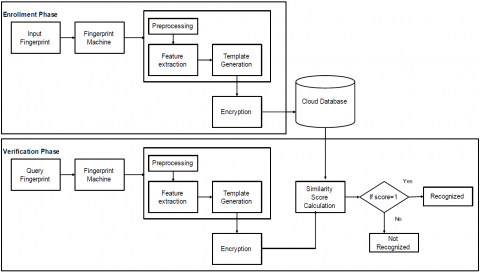Figure 1. Proposed system block diagram

3.1 Enrollment phase

In the enrollment phase, shown in Figure. 2, an individual user fingerprint is enrolled and stored in the database by fingerprint detection machine. After the storage of an individual's image, its quality is checked and if the quality level is appropriate then feature extraction is done. The proposed system uses the minutiae point algorithm  for feature extraction. Minutiae points are very important and widely used features of the fingerprint detection technique. These are used for matching an appropriate fingerprint with stored templates of fingerprints in the database. Minutiae points are used to distinguish one fingerprint image from others. A fingerprint image with good quality can have 25 to 85 minutiae points . These minutiae points are individualities in the finger ridge patterns of an individual. In this, the two most widely used features are ridge ending and ridge bifurcation. Ridge ending is the sudden end point of the ridge while ridge bifurcation is the point where two or more branches are generated from the single ridge shown in Figure 3. For extraction of minutiae points the binary image-based method is used. This method requires to convert each grayscale pixel to the binary values 0 or 1.

$I(x, y)=\left\{\begin{array}{l}{1, \text {if }(x, y) \geq t} \\ {0, \text { Otherwise }}\end{array}\right.$        (1)

## 2.pngFigure 2. Enrollment phase

## 3.jpgFigure 3. Ridge ending and ridge bifurcation

After the conversion of a binary image, it goes through morphological thinning, which reduces ridge to one pixel of thickness for minutiae extraction. In the thinned binary image each pixel (p) is analyzed to find the location of minutiae. This is achieved by Eq. 2, called a Rutovitz crossing number .

$c n(p)=\frac{1}{2} \sum_{i=1 . .8}\left|v a l\left(p_{i m o d 8}\right)-v a l\left(p_{i-1}\right)\right|$         (2)

where val is 0 or 1. Minutiae locations ridge ending is now identified as cn=1 and ridge bifurcations are cn=3.

There are four parameters that are used to describe each detected minutiae point. Let ith minutiae point is represented as Mi.

$M_{i}=\left(x_{i}, y_{i}, \theta_{i}, T_{i}\right)$

where minutiae point coordinates are xi and yi, θi is the direction of inutiae, Ti is the called a type of minutiae point whether ridge ending or bifurcation.

Further, minutiae points are a most accepted feature in fingerprint but, the pre-processing of the image does not eliminate all the defects from the original grey level image. For that, we require some other approach to remove false minutiae points caused by poor quality. We have applied the Principal Component Analysis (PCA) technique for improving the recognition rate of fingerprints .

3.1.1 Principal component analysis

PCA is a statistical approach that translates an image of MxN to a vector according to the rows and columns. Therefore, the image of size M x N becomes a vector of dimension (D). PCA is used as a tool for the reduction of multidimensional data to low dimensions. The basic steps of PCA are shown in Fig. 6. The main advantage of using the PCA technique is the reduction of complexity in images and also reduction of noise level because of choosing a maximum variation basis, so the background small variations are ignored automatically.

## 4.png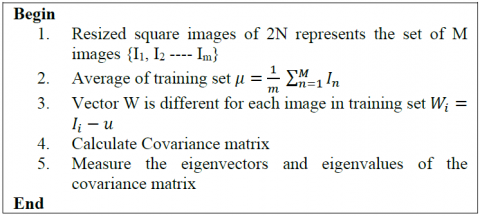Figure 4. Steps of PCA

3.1.2 Encryption of fingerprint

This is the second step in the proposed system for adding more security for individual user identification. In this Elliptic curve encryption with a digital signature algorithm is applied for encryption of fingerprint templates. It is public key cryptography, which is based on the algebraic structure of elliptic curve over finite fields. An elliptic curve is shown in Fig. 7 and equations of the elliptic curve are given as 3 and 4.

$y^{2}=x^{3}+a x+b$         (3)

$4 a^{3}+27 b^{2} 6=0$        (4)

A pair (x,y), where $x, y \in F_{p}$ , is a point on the elliptic curve, if (x,y) satisfies the Eq. 3. The point at infinity is also said to be on the same curve. The set of all the points on the elliptic curve (E) is denoted by E (Fp). Let E be an elliptic curve defined over a finite field Fp. Let P be a point in E (Fp), and suppose that P has prime order n. Then the cyclic subgroup of E (Fp) generated by P is-

$<P>=\{\infty, P, 2 P, 3 P, \ldots . .,(n-1) P\}$          (5)

The prime p, the equation of the elliptic curve E, and the point P and its order n are the public domain parameters. There are three operations done on an elliptic curve.

## 5.jpgFigure 5. Elliptic curve

3.1.3 Point multiplication

In this operation, a new point Q can be obtained on the same elliptic curve by multiplying a point P on the elliptic curve and is with scalar k, kP=Q. To obtain point multiplication two basic operations are used-

Point addition it is an operation of adding two points to obtain another point.

$R=P+Q,$ if $P 6=Q$

Point doubling It is an operation in which a new point is obtained by adding a point P by itself.

$R=2 P$ if $P+Q$ then $P+Q=2 P$

3.1.4 Point subtraction

Let P and Q are two distinct points, such that

$P=\left(x_{j}, y_{j}\right), Q=\left(x_{b} y_{k}\right),$ then $P-Q=P+(-Q),$ where $Q=\left(x_{b}-y_{k} \bmod p\right)$

3.1.5 Multiplicative inverse

Let Fp is a finite field, if $x \in F_{p}$  such that ax ≡ 1( mod p), ax = 1 in Fp , x is called multiplicative inverse of a, can be represented by a−1 mod p.

The main advantage of using elliptic curve cryptography is that it uses smaller keys in comparison to other cryptographic algorithms for very fast key generation. At the time of enrollment of fingerprint, the system takes an input image from which features are extracted. After the extraction of the features, the fingerprint template is encrypted using elliptical encryption. The identification of the user is done by similarity matching between query and stored image templates. The encrypted test fingerprint templates are matched with stored database templates [24-25].

In the proposed method, the coefficients of the elliptic curve are generated from minutiae points. Before the encryption process, there is a need to generate private and public keys. Following are the steps to generate private and public keys shown in Algorithm 2 shown in Figure. 6. After the generation of keys, a digital signature is generated. Using a digital signature recipient of a message can verify the message authenticity using a public key. For this, a secure hash algorithm is used to convert the variable-length message into a fixed-length message called as digest h(m), signature generation is shown as Algorithm -3, shown in Figure. 7. After the generation of the signature, it needs to be verified for the query message for its authenticity using the public key. Signature verification algorithm in Algorithm -4, shown in Figure. 8. The system uses Paillier Homomorphic encryption for storing and accessing data in the cloud for more security and privacy. If the user is authorized then he can access data from the cloud. But in the system, the data stored in the cloud is also encrypted using the Paillier encryption algorithm . The Paillier algorithm has a unique feature of additive homomorphic property shown in Eq. 6. The whole communication in the proposed system is done in encrypted form only.

Encrypt $(P 1 \oplus P 2)=$ Encrypt $(P 1) \oplus$ Encrypt $(P 2)$       (6)

## 6.pngFigure 6. Generation of keys

## 7.png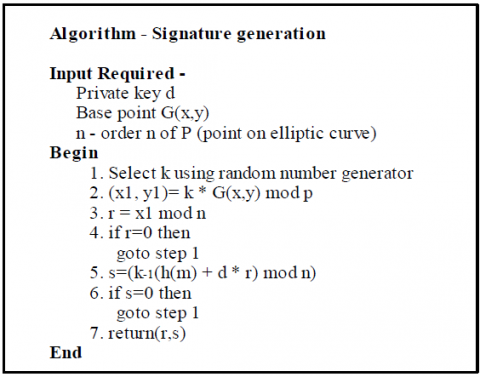Figure 7. Steps of signature generation

## 8.png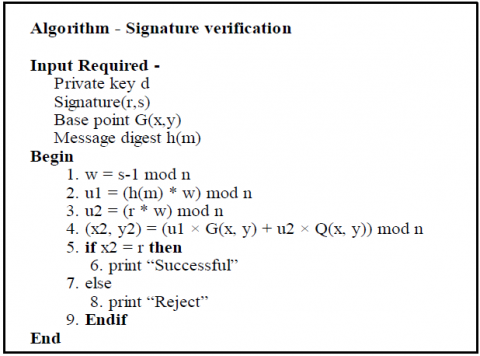Figure 8. Steps of signature verification

3.2 Verification phase

After enrollment of all the fingerprint of authorized users, verification will be done each time a user wishes to access cloud data. The verification process is shown in Figure. 9 Verification process is done by extracting minutiae points of a user, who wants to access the cloud system. After the extraction of the features, the matching score also called a similarity score is calculated for the query image with each template existent in the database. This similarity score describes the level of similarity between two fingerprints. The matching process Algorithm is shown in Figure. 10, which compares both minutiae point sets viz. Input image I= m1, m2, .m3- mi) and template stored in database T= m1, m2, m3 - mj). The algorithm then returns a similarity score of T and I represented by S (I, T). Two minutiae points are called as matched points if the calculated difference of position and direction are less then acceptance distances. Let A and B are two images, where A is a sample image from the dataset and B is the query image. A and B is a set of minutiae points represented by Eq. 6 and 7 respectively. The position between A and B is represented as a geometric distance in Eq. 8 and minutiae angle difference in Eq. 9.

## 9.pngFigure 9. Verification Phase

$A=\left(m_{A 1}, m_{A 2}, \ldots \ldots, m_{A p}\right)$         (6)

where $m_{A i}=\left(x_{A i} y_{A i} \theta_{A i}\right)$ and $1 \leq i \leq p$

$B=\left(m_{B 1}, m_{B 2}, \ldots \ldots, m_{B q}\right)$          (7)

where $m_{B j}=\left(x_{B j}, y_{B j}, \theta_{B j}\right)$ and $1 \leq j \leq q$

$\operatorname{Dist}_{r}\left(m_{A i}, m_{B j}=\sqrt{\left(x_{A i}-x_{B j}\right)^{2}+\left(y_{A i}-y_{B j}\right)^{2}<r_{a}}\right.$     (8)

$\operatorname{Dist}_{\theta}\left(m_{A i}, m_{B j}=\min \left(\left|\theta_{A i}-\theta_{B j}\right|, 360^{\circ}-\left|\theta_{A i}-\theta_{B j}\right|\right)<r_{\alpha}\right.$     (9)

## 10.pngFigure 10. Calculation of Similarity Score

4. Results and Discussion

4.1 Experiment setup

The simulations of the proposed work are done in MATLAB R2015a using a workstation with Intel coreTM i3 3.2 GHz processor. The proposed approach has been applied on the dataset of 300 fingerprint grayscale images, captured from the employees of a University situated in north India. For the researcher's comfort, the collected images have been resized to a square image of size 300×300. To measure the performance of the system, we have split the database into two parts- training and testing. For training of the system 80% of the data has been used. For testing, 20% of data has been chosen from the dataset.

4.2 Performance evaluation and comparison

The minutiae point algorithm is used for matching the fingerprint and finding the similarity score for the individual users. For generating image template original image is converted into masked, thin and then minutiae-points image generated Figure. 11 shows the masked, thinned and minutiae-point generated images along with four sample input images chosen from the database. Figure. 12 displays the similarity score after matching the minutia points of query and two template images taken from the cloud database. Three verification metrics namely, False Matching Ratio (FMR), False Non Matching Ratio (FNMR), and Recognition Rate (RR) have been determined. FMR determines a probability at which any system incorrectly predicts the unauthorized biometric entity as a correct entity, while FNMR is the probability at which any system predicts the right entity as wrong. The equation of FMR and FNMR has been shown in Eq. 10 and 11 and the plots of FMR and FNMR of the sample query image have been shown in Figure. 13. From Figure. 13, it can be clearly observed that the FMR and FNMR rate getting better when repeated for 100 iterations. Equal Error Rate (EER) is approximately 0.38 where FMR and FNMR value are equal. After applying the PCA algorithm the system produces approximately 97% of accuracy, shown in Table 1 and the time is taken in the encryption of biometric features used for recognition has been shown in Table 2.

$F M R=\frac{\text {FalseMatches}}{\text {Imposter Attempts}}$       (10)

$F N M R=\frac{\text {False} N \text {onMatches}}{\text {EnrolledAttempts}}$        (11)

## 11.png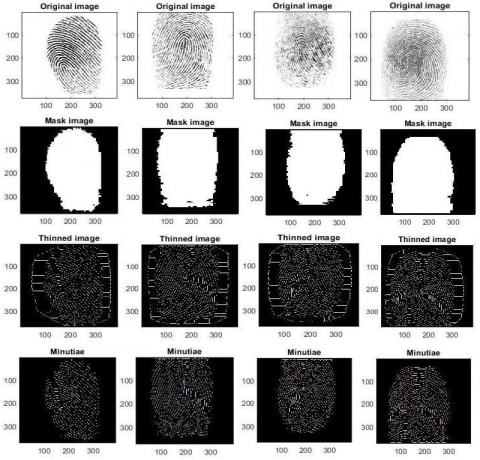Figure 11. Mask, thinned and minutiae points for the four original input images

## 12.png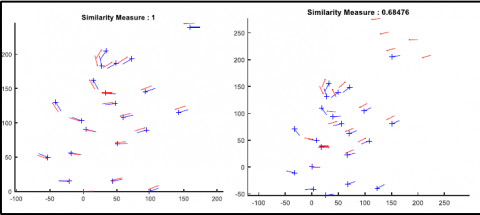Figure 12. Matching query and template image with similarity score 1 and Unmatched query and template image with similarity

## 13.jpg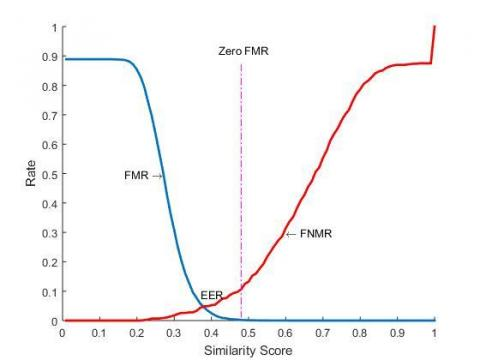Figure 13. Plots of FMR and FNMR

Table 1. Recognition accuracy of individual images

 Sno. Image No. Encrypted Template Time (s) Performance 1 101 68,348,174,166 13 92.11 2 102 12,495,642,070 15 94.67 3 109 24,579,125,485 18 95.57 4 110 13,785,953,190 22 97

Table 2. Computation time for verification of individual images

 Sno. User Image Image size (pixels) Time Taken (s) 1 101 60 x 60 180 2 102 100 x 100 220 3 109 200 x 200 275 4 110 250 x 250 297

To validate the performance of the proposed system, we have compared our approach with some existing approaches given by various researchers such as Haghighat et al. , Kumar et al. , Shakil et al. , and Balton et al.  etc. In the research study proposed by Haghighat et al. , a cloud system based biometric recognition for individual user authentication has been presented. The system adds the details of users to their biometric and store it after encryption. The facial images are used as biometric. The system also uses the Generalized Local Discriminant Analysis (GLDA) to classify

extracted features. The system has claimed 95% recognition accuracy. In the study done by Kumar et al. , the authors proposed a Biometric Face Recognition (BFR) system using face detection. The authors have used the Eigenface detection algorithm with the encryption using the elliptic curve. The system claimed 96.89% recognition accuracy. Shakil et al. , illustrated the cloud-based system for healthcare. The system ensures the privacy and security of electronic medical data. The system uses a signature-based biometric authentication system. The authors have used the Back Propagation Neural network (BPN) for the training of signatures data. The system claimed sensitivity of 0.98 and a specificity of 0.95. The study proposed by Balton et al. , a cloud biometric system has been presented for authentication. The system uses fingerprint and iris codes as biometric. The system uses Minutiae points and Iris codes (MI) for feature extraction. The system claimed to consume the total time for offline detection 3178 + 79.5/fused features. The summary table of comparisons is shown in Table. 3, the accuracy plot is shown in Figure. 14, After done the comparison to all the above mentioned studies here, the proposed system has achieved an accuracy of 97%, which is showing a slight improvement over others. But given the cost and advantages over other biometrics systems as mentioned in the above studies, the proposed system is better than the existing approaches. Figure. 15, shows the recognition rate comparison plot between the proposed approach and other works. The figure shows clearly that the recognition rate of proposed work is far better than other stated approaches in terms of time to recognize query fingerprint.

## 14.png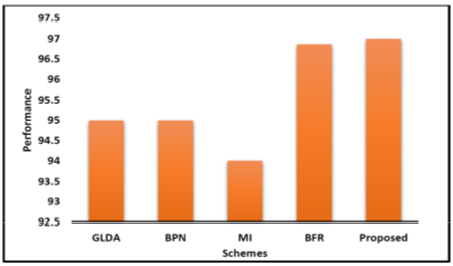Figure 14. Plot of performance comparison

## 15.pngFigure 15. Plots of recognition rate comparison

Table 3. Comparative analysis of existing biometric systems

 Sno. Study Biometric Approach Used Performance 1 GLDA Facial images GLDA Recognition accuracy -95 % 2 BFR Face images Eigen face, encryption Recognition accuracy-96.89 % 3 BPN Signatures Backpropagation neural network Sensitivity 0.98, specificity 0.95. 4 MI Fingerprint and iris codes Minutiae points and iris codes 3178 +79.5 offline detection time 89 + 149.2 online detection time 5 Proposed Study Fingerprints and encryption with digital signature and homomorphic encryption Minutiae points, PCA , Hybrid two layer encryption Recognition accuracy 97 %
5. Conclusion and Future Scope

In this paper, a secure and privacy-preserving cloud system has been proposed, which is based on a hybrid biometric recognition system and elliptic curve cryptography. The system identifies cloud users according to their encrypted fingerprint templates stored in the encrypted domain. For feature extraction, a minutiae point detection algorithm is used which uses two features ridge ending and ridge bifurcations. The query image can be recognized according to the proposed algorithm which generates a similarity score in terms of FMR and FNMR which lies between 0 to 1. To improvise the recognition accuracy by reducing the noise PCA approach has been applied to the proposed system. After experimental evaluation of the proposed scheme, it has been found that the system recognition accuracy is approximately 97% which is quite better than state-of-art recent approaches. The main shortcoming of the system is the storage requirement. As the system goes in real time, the database size requirement gets increased significantly because of the large size of images in comparison to traditional authentication data. Further, a small dataset has been chosen for testing purpose which can be taken into consideration in the future. Also, a combination of one or more traditional features or biometric parameters like passwords, retina scan, signature, etc. can be added to make the system more robust and secure.

References

 Fiandrotti, A., Mattelliano, M., Baccaglini, E., Vergori, P. (2018). CDVSec: Privacy-preserving biometrical user authentication in the cloud with CDVS descriptors. Pattern Recognition Letters, 113: 67-74. https://doi.org/10.1016/j.patrec.2017.03.024

 Jain, A.K., Ross, A.A., Nandakumar, K. (2011). Introduction to biometrics. Springer Science & Business Media. https://doi.org/10.1007/978-0-387-77326-1

 Ratnam, S., Gupta, M., Singh, D.A.S. Thirunavukkarasu, K. (2016). A survey on biometric security technologies from cloud computing perspective. International Journal of Scientific and Technology Research, 4(8): 22–24.

 Jain, A.K., Pankanti, S., Prabhakar, S., Hong, L., Ross, A., Wayman, J.L. (2004, August). Biometrics: A grand challenge. Proceedings of the 17th International Conference on Pattern Recognition, Cambridge, UK, pp. 935-942. https://doi.org/10.1109/ICPR.2004.1334413

 Jain, A.K., Ross, A., Pankanti, S. (2006). Biometrics: A tool for information security. IEEE transactions on Information Forensics and Security, 1(2): 125-143. https://doi.org/10.1109/TIFS.2006.873653

 Jain, P., Rane, D., Patidar, S. (2011). A survey and analysis of cloud model-based security for computing secure cloud bursting and aggregation in renal environment. In 2011 World Congress on Information and Communication Technologies, IEEE, Mumbai, India, pp. 456-461. https://doi.org/10.1109/WICT.2011.6141288

 Gupta, B., Agrawal, D.P., Yamaguchi, S. (2016). Handbook of Research on Modern Cryptographic Solutions for Computer and Cyber Security. IGI Global. https://doi.org/10.4018/978-1-5225-0105-3

 Bhattasali, T., Saeed, K., Chaki, N., Chaki, R. (2015). A survey of security and privacy issues for biometrics based remote authentication in cloud. In IFIP International Conference on Computer Information Systems and Industrial Management, Springer, Berlin, Heidelberg, pp. 112-121. https://doi.org/10.1007/978-3-662-45237-0_12

 Naveed, G., Batool, R. (2015). Biometric authentication in cloud computing. Journal of Biometrics & Biostatistics, 6(5): 1. https://doi.org/10.4172/2155-6180.1000258

 Haghighat, M., Zonouz, S., Abdel-Mottaleb, M. (2015). CloudID: Trustworthy cloud-based and cross-enterprise biometric identification. Expert Systems with Applications, 42(21): 7905-7916. https://doi.org/10.1016/j.eswa.2015.06.025

 Hahn, C., Hur, J. (2016). Efficient and privacy-preserving biometric identification in cloud. ICT Express, 2(3): 135-139. https://doi.org/10.1016/j.icte.2016.08.006

 Bala, Y., Malik, A. (2018). Biometric inspired homomorphic encryption algorithm for secured cloud computing. In Nature Inspired Computing, Springer, Singapore, pp. 13-21. https://doi.org/10.1007/978-981-10-6747-1_2

 Pan, S., Yan, S., Zhu, W.T. (2016, July). Security analysis on privacy-preserving cloud aided biometric identification schemes. In Australasian Conference on Information Security and Privacy, Springer, Cham, pp. 446-453. https://doi.org/10.1007/978-3-319-40367-0_29

 Kumar, S., Singh, S.K., Singh, A.K., Tiwari, S., Singh, R.S. (2018). Privacy preserving security using biometrics in cloud computing. Multimedia Tools and Applications, 77(9): 11017-11039. https://doi.org/10.1007/s11042-017-4966-5

 Lee, P. (2017). Prints charming: how fingerprints are trailblazing mainstream biometrics. Biometric Technology Today, 2017(4): 8-11. https://doi.org/10.1016/S0969-4765(17)30074-7

 Zhang, C., Zhu, L., Xu, C. (2017). PTBI: An efficient privacy-preserving biometric identification based on perturbed term in the cloud. Information Sciences, 409: 56-67. https://doi.org/10.1016/j.ins.2017.05.006

 Kumari, S., Li, X., Wu, F., Das, A.K., Choo, K.K.R., Shen, J. (2017). Design of a provably secure biometrics-based multi-cloud-server authentication scheme. Future Generation Computer Systems, 68: 320-330. https://doi.org/10.1016/j.future.2016.10.004

 Al-Hamami, A.H., AL-Juneidi, J.Y. (2015). Secure mobile cloud computing based-on fingerprint. World of Computer Science & Information Technology Journal, 5(2): 23-27.

 Shakil, K.A., Zareen, F.J., Alam, M., Jabin, S. (2017). BAMHealthCloud: A biometric authentication and data management system for healthcare data in cloud. Journal of King Saud University-Computer and Information Sciences. https://doi.org/10.1016/j.jksuci.2017.07.001

 Maltoni, D. (2003). A tutorial on fingerprint recognition, advanced studies in biometrics. Summer School on Biometrics, Alghero, Italy. https://doi.org/10.1007/11493648_3

 Wieclaw, L. (2009). A minutiae-based matching algorithms in fingerprint recognition systems. Journal of Medical Informatics & Technologies, 13.

 Rutovitz, D. (1966). Pattern recognition, in proceedings of journal in Royal Statistical Society, vol. 129, ser. https://doi.org/10.2307/2982255

 Wang, Y.X., Ao, X.Y., Du, Y.F., Li, Y.P. (2006). A fingerprint recognition algorithm based on principal component analysis. In TENCON 2006-2006 IEEE Region 10 Conference, Hong Kong, China, pp. 1-4. https://doi.org/10.1109/TENCON.2006.344032

 Martinez, V.G., Encinas, L.H., Ávila, C.S. (2010). A survey of the elliptic curve integrated encryption scheme. Ratio, 80(1024): 160-223.

 Shankar, T.N., Sahoo, G., Niranjan, S. (2012). Using the digital signature of a fingerprint by an elliptic curve cryptosystem for enhanced authentication. Information Security Journal: A Global Perspective, 21(5): 243-255. https://doi.org/10.1080/19393555.2012.694978

 Paillier, P. (1999, May). Public-key cryptosystems based on composite degree residuosity classes. In International Conference on the Theory and Applications of Cryptographic Techniques, Berlin, Heidelberg, pp. 223-238. https://doi.org/10.1007/3-540-48910-X_16

 Blanton, M., Gasti, P. (2011). Secure and efficient protocols for iris and fingerprint identification. In European Symposium on Research in Computer Security, Springer, Berlin, Heidelberg, pp. 190-209. https://doi.org/10.1007/978-3-642-23822-2_11